Mathematical and Physical Journal
for High Schools
Issued by the MATFUND Foundation
 Already signed up? New to KöMaL?

# KöMaL Problems in Physics, May 2013

Show/hide problems of signs:## Problems with sign 'M'

Deadline expired on June 10, 2013.

M. 333. The temperature of air in a small flask, which is covered by soot, is measured with a thermometer. Place the flask next to a filament lamp, rated at a high power, and measure how the temperature depends on the distance from the lamp.

(6 pont)

statistics## Problems with sign 'P'

Deadline expired on June 10, 2013.

P. 4540. 1 kg ice at a temperature of -30 oC is placed into a thermally insulated vessel filled with water of temperature 30 oC. Is it possible that no phase change occurs?

(3 pont)

solution (in Hungarian), statistics

P. 4541. How long is the shadow of a 1 m long rod, which is fixed perpendicularly to the ground at the equator,

a) at noon, on the 21-st of June;

b) 2 hours later after noon on the 21-st of June?

(5 pont)

solution (in Hungarian), statistics

P. 4542. Find the equivalent resistance of the resistor system shown in the figure (between the two endpoints) if the resistance of each the resistor is R.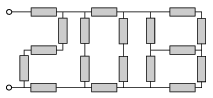(4 pont)

solution (in Hungarian), statistics

P. 4543. When blowing soap bubbles, it can often be seen that the colourful soap bubbles lose their colour and darken just before they burst. What is the reason for this phenomenon?

(4 pont)

solution (in Hungarian), statistics

P. 4544. Using an electronic kitchen scale, which shows grams, the same 1 g is gained for the weights of a rubber, a pencil and a pen. The pencil and the pen together weigh 2 grams, and the total weight of the pencil and the rubber is also 2 grams. Measuring the three items together the scale reads 3 grams. The total weight of the pen and the rubber is also 3 grams. How can this happen? What can the range of the mass of each item be? What can be stated about the total mass of the three items?

(5 pont)

solution (in Hungarian), statistics

P. 4545. Two cylinder shaped vessels of cross section A are fixed vertically to a trolley, which was initially at rest. The two vessels are connected with a thin horizontal tube which is equipped with a tap. The distance between the axes of the vessels is L. The vessel in the left hand side is filled with some liquid of density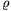; and at this time the total mass of the system at rest is m.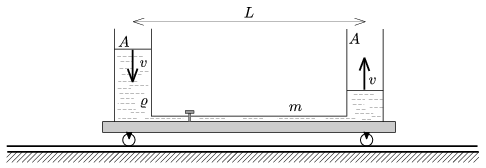After opening the tap what is the speed of the cart at the moment when the speed of the water levels in the vessels is v? (Rolling friction, friction in the bearings and air-drag are negligible.)

(5 pont)

solution (in Hungarian), statistics

P. 4546. The top part of a thermally insulated, vertical cylinder of cross section A=0.5dm2 is fixed. There is a sample of Helium gas in the cylinder and initially its pressure is p0=105 Pa, its length is0=50 cm. The bottom part of the cylinder is closed with an initially fixed thermally insulating piston of mass m=10 kg. The external air pressure is also p0. Then the piston is released, and it can move frictionlessly in the cylinder.

a) What is the length of the helium confined in the cylinder when the speed of the piston is maximum?

b) What is the maximum speed of the piston?

c) What is the greatest length of the confined gas?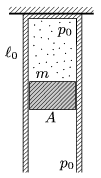(5 pont)

solution (in Hungarian), statistics

P. 4547. A simple pendulum of lengthis hanging above the horizontal, grounded metal floor at a height of. Its period is T1=2.00 s. How much charge was added to the bob of mass m=25 gram, if the period of the pendulum decreased to T2=1.96 s?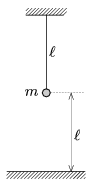(4 pont)

solution (in Hungarian), statistics

P. 4548. A metal sphere is initially charged to a potential difference of U0, and is hung by a totally insulating thread. Then it is left in the air of resistivity. How long does it take that the voltage of this condenser decreases to U0/2?

(5 pont)

solution (in Hungarian), statistics

P. 4549. A thyristor is an electrical element made of semiconductor, and can be used in many different ways. Its current-voltage characteristics is quite strange. (See figure 1.)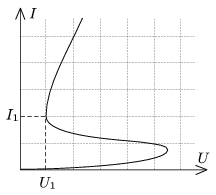The thyristor is connected as shown in figure 2, and the voltage U0 is slowly increased from zero to Umax , and then it is slowly decreased back to zero.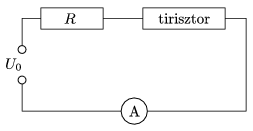Graph the current as a function of the voltage U0, if

a)and,

b)and.

(5 pont)

solution (in Hungarian), statistics

P. 4550. Two alike long, straight wires of diameter d, are at a distance of; their resistance is negligible and (L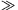D). One ends of the wires are connected through a battery of voltage U, and the other ends of the wires are connected through a resistor of resistance R. What is the value of R if the electric and magnetic forces exerted between the wires are equal?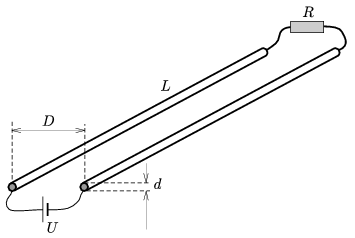(6 pont)

solution (in Hungarian), statistics